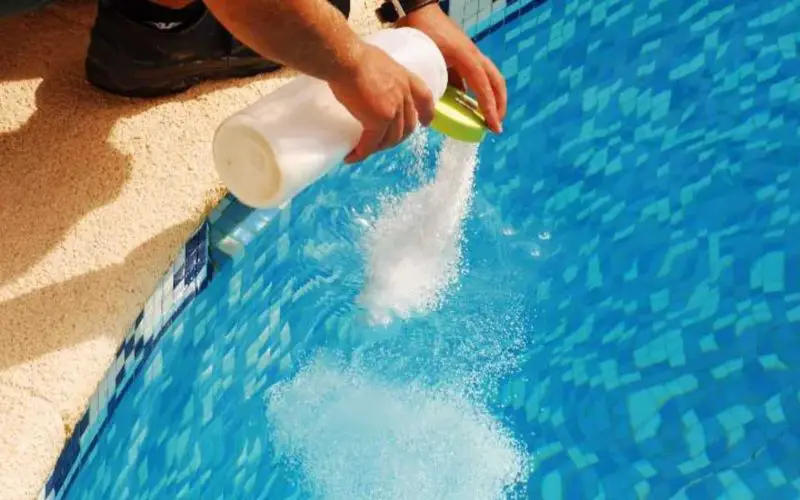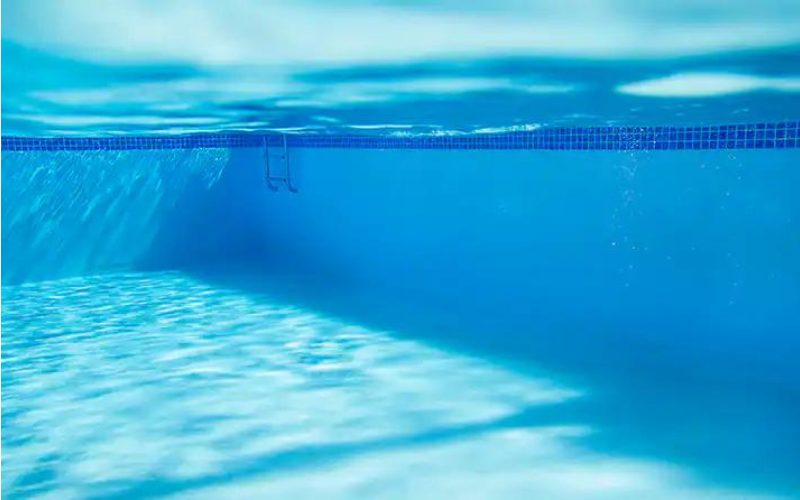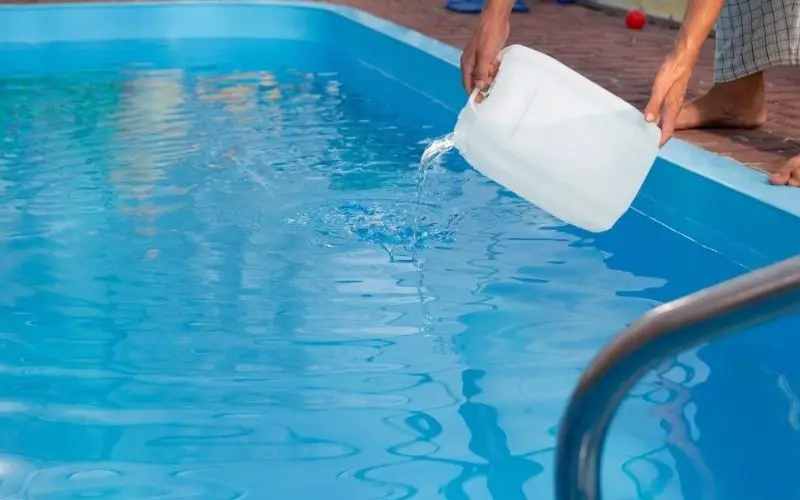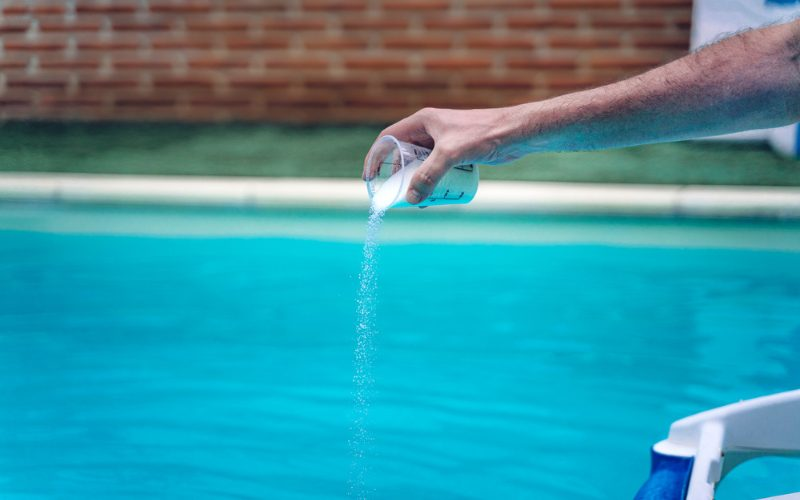# How much liquid chlorine is to add to the pool?

If you have a pool in your yard, liquid chlorine is compulsory to disinfect the water. Chlorine is necessary because it is a disinfectant that kills germs that may spread water-borne diseases.

However, it is essential to know the quantity of liquid chlorine to add to your pool, as a small quantity is ineffective, and a large quantity is dangerous.

Contents

## Determining if your pool needs chlorineThe last thing you want is to add chlorine to water containing, so it is essential to know if your pool needs chlorine. One fundamental way you can get this information is by using a pH and chlorine testing kit to determine the pH of the water and the amount of chlorine contained.

The ideal pH for any swimming pool is between 7.4 and 7.6. At this pH level, the water is safe for people to swim inside and provides a medium that allows chlorine to kill germs effectively. Your chlorine level should be between the range of 1 – 3 parts per million (ppm).

After obtaining the testing kits, follow the instructions to determine the chlorine level you need for your pool.If you have identified that your pool is low on chlorine, then the following action you need is to calculate the amount of chlorine you need to keep the pool and its users safe when swimming. The primary process involved in this stage is calculating the volume of your pool. The results give you an estimate of how much chlorine you need.

### Calculating the volume of your pool

If, before installing your in-ground or above-ground pool, you have been notified of the volume of your pool, then you can proceed to the next stage. You will need some of the following details to get the volume of your pool or how many gallons it can hold.

1. Length
2. Width
3. Height
4. Depth
5. Area
6. Diameter
8. Pi (π)

The details you need depend on the shape of your pool, but overall, one cubic meter holds about 7.5 gallons of water.

#### Rectangle pool

If you have a rectangle pool, you must measure the length, the width ( the longest side), and the depth (how far into the ground the pool extends). After gathering these details, you should have three values, and to find the volume of your rectangular pool, use the formula V = L × W × H. The result of this multiplication gives you the volume in cubic feet. To know how many gallons your pool can hold, multiply your result by 7.5.

So it becomes V = (L × W × H) × 7.5

If your pool has a gradual slope moving downwards, you can obtain the depth by measuring the shallow and deep sides and then taking their average, which should be divided by two.

#### Round pool

Using a measuring tape, measure the distance between one end of your circular pool to the other end. The measurement you obtain from this is called the diameter. You can further obtain the radius [r] by dividing the diameter by two. After getting the pool’s radius, calculate the area using the formula A = πr².

After obtaining the area, measure the depth of your pool and multiply this value by the area. As always, your volume is in cubic feet, and to determine what it is in gallons, multiply the volume by 7.5.

To calculate the quantity of chlorine you need for the pool, you need to multiply the volume of your pool in gallons by 0.00013. For example, if your pool contains 12,000 gallons of water, then to find the amount of chlorine needed for an increase, you can calculate it by:

12,000 × 0.00013 = 1.56 ounces

This formula allows you to know the exact quantity of chlorine you need if you want to increase the existing composition in your pool by 1.How often do you need to add chlorine to your pool water for maximum safety against diseases? The answer to this question depends on several factors we will explain briefly.

### Pool usage

A general rule is that the more a pool is used, the more often it needs chlorine. That means that if you use your pool once a week, you must use your chlorine test kit once. However, if the pool is used regularly, you must test the chlorine level 2 – 3 times weekly to determine if it is short of this disinfectant.

### Dirt

It is normal to find dirt and debris in your pool. But if there is too much dirt and debris, there is a high possibility that the disinfecting power of the chlorine has been exhausted. If needed, you must test and supplement the pool water with chlorine.

### Sunlight

Excess sunlight affects chlorine by breaking it down or disintegrating the molecules. The reducing property of sunlight reduces the effectiveness of this disinfectant. If your pool has been exposed to sunlight for a long time, you must replenish the amount of chlorine available.

To prevent this, you can opt for using pool covers to minimize the effects of sunlight on your pool.

## Conclusion

Adding chlorine to your pool is essential to ensure minimal water-borne diseases. However, it can get confusing if you need to know the appropriate quantity of liquid chlorine for your pool. After reading this article, you should be able to test your water and supplement it with chlorine when needed.

You May Also Like:

The amount of chlorine you need depends on the volume or capacity of your pool. We have provided a brief guide on determining the volume of your pool and calculating the amount of chlorine necessary for clean and healthy water.